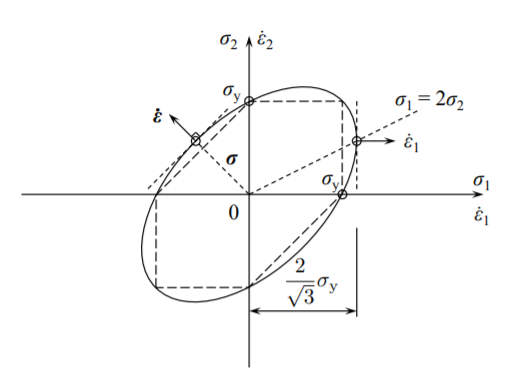# 11.6: Flow Rule

$$\newcommand{\vecs}{\overset { \rightharpoonup} {\mathbf{#1}} }$$ $$\newcommand{\vecd}{\overset{-\!-\!\rightharpoonup}{\vphantom{a}\smash {#1}}}$$$$\newcommand{\id}{\mathrm{id}}$$ $$\newcommand{\Span}{\mathrm{span}}$$ $$\newcommand{\kernel}{\mathrm{null}\,}$$ $$\newcommand{\range}{\mathrm{range}\,}$$ $$\newcommand{\RealPart}{\mathrm{Re}}$$ $$\newcommand{\ImaginaryPart}{\mathrm{Im}}$$ $$\newcommand{\Argument}{\mathrm{Arg}}$$ $$\newcommand{\norm}{\| #1 \|}$$ $$\newcommand{\inner}{\langle #1, #2 \rangle}$$ $$\newcommand{\Span}{\mathrm{span}}$$ $$\newcommand{\id}{\mathrm{id}}$$ $$\newcommand{\Span}{\mathrm{span}}$$ $$\newcommand{\kernel}{\mathrm{null}\,}$$ $$\newcommand{\range}{\mathrm{range}\,}$$ $$\newcommand{\RealPart}{\mathrm{Re}}$$ $$\newcommand{\ImaginaryPart}{\mathrm{Im}}$$ $$\newcommand{\Argument}{\mathrm{Arg}}$$ $$\newcommand{\norm}{\| #1 \|}$$ $$\newcommand{\inner}{\langle #1, #2 \rangle}$$ $$\newcommand{\Span}{\mathrm{span}}$$$$\newcommand{\AA}{\unicode[.8,0]{x212B}}$$

The simplest form of the associated flow rule for a rigid perfectly plastic material is given by

$\dot{\epsilon}_{ij} = \dot{\lambda} \frac{\partial F(\sigma_{ij})}{\partial \sigma_{ij}}$

where the function $$F(\sigma_{ij})$$ is defined by Equation (11.3.8), and $$\dot{\lambda}$$ is the scalar multiplication factor. Equation (11.3.11) determines uniquely the direction of the strain rate vector, which is always directed normal to the yield surface at a given stress point. In the case of plane stress, the two components of the strain rate vector are

$\dot{\epsilon}_1 = \dot{\lambda} (2\sigma_1 − \sigma_2)$

$\dot{\epsilon}_2 = \dot{\lambda} (2\sigma_2 − \sigma_1)$

The magnitudes of the components $$\dot{\epsilon}_1$$ and $$\dot{\epsilon}_2$$ are undetermined, but the ratio, which defines the direction $$\dot{\epsilon}/\epsilon_2$$, is uniquely determined.

In particular, under the transverse plain strain $$\dot{\epsilon}_2 = 0$$, so $$\sigma_1 = 2\sigma_2$$ and $$\sigma_1 = \frac{2}{\sqrt{3}} \sigma_y$$.Figure $$\PageIndex{1}$$: The strain rate vector is always normal to the yield surface.

This page titled 11.6: Flow Rule is shared under a CC BY-NC-SA 4.0 license and was authored, remixed, and/or curated by Tomasz Wierzbicki (MIT OpenCourseWare) via source content that was edited to the style and standards of the LibreTexts platform; a detailed edit history is available upon request.